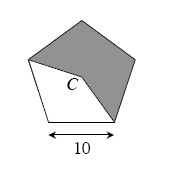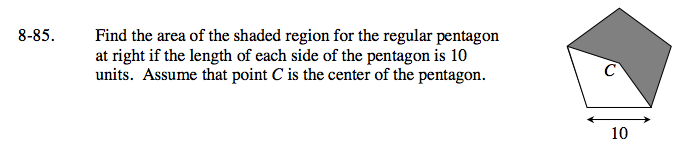Home > GC > Chapter 8 > Lesson 8.3.1 > Problem8-85

8-85.Find the area of the shaded region for the regular pentagon at right if the length of each side of the pentagon is 10 units. Assume that point C is the center of the pentagon. Homework Help ✎Try dividing the pentagon into congruent triangles. What trig ratio can you use to find the area of one of these triangles?
Use the area of one triangle as a starting point to find the area of the shaded region.